﻿ 相对论的德布罗意物质波及其应用于量子跃迁Rabi振荡过程 Relativistic De Broglie Matter Wave and Its Application to Dynamic Rabi Flopping in Quantum Transition

Modern Physics
Vol.08 No.03(2018), Article ID:25097,9 pages
10.12677/MP.2018.83017

Relativistic De Broglie Matter Wave and Its Application to Dynamic Rabi Flopping in Quantum Transition

Huaiyang Cui

Department of Physics, Beihang University, Beijing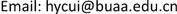Received: May 4th, 2018; accepted: May 21st, 2018; published: May 28th, 2018ABSTRACT

A new approach is used for extending the De Broglie matter wave to relativistic formalism. The relativistic matter wave expression is obtained, and successfully applied to analyze the dynamic process of Rabi flopping in atomic quantum transition. Under external electromagnetic wave, the electronic probability flow in an atom becomes an alternating current, positively responding to the external electromagnetic wave. In the resonance process, the atomic alternating current absorbs or emits the electromagnetic wave energy by the amount of one photon energy. The resonance condition is just the selection rule. The dynamic evolution curve was calculated by computer simulation.

Keywords:Quantum Transition, Alternating Current, Selection Rule1. 引言

2. 相对论的德布罗意物质波

$\begin{array}{l}m\frac{\text{d}{u}_{A\mu }}{\text{d}\tau }=q{F}_{\mu \nu }{u}_{A\nu }\\ m\frac{\text{d}{u}_{B\mu }}{\text{d}\tau }=q{F}_{\mu \nu }{u}_{B\nu }\end{array}$ (1)

$m\frac{\text{d}{u}_{\mu }}{\text{d}\tau }=m\frac{\partial {u}_{\mu }}{\partial {x}_{\nu }}\frac{\text{d}{x}_{\nu }}{\text{d}\tau }=m{u}_{\nu }\frac{\partial {u}_{\mu }}{\partial {x}_{\nu }}$ (2)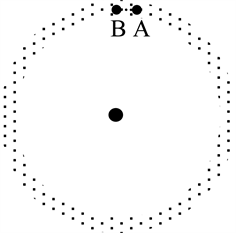Figure 1. The electrons at A and B in the hydrogen atomic electron cloud are governed by the same dynamics, and different in phase for their matter waves

${u}_{\nu }\frac{\partial \left(m{u}_{\mu }\right)}{\partial {x}_{\nu }}=q{F}_{\mu \nu }{u}_{A\nu }={u}_{\nu }\left[\frac{\partial \left(q{A}_{\nu }\right)}{\partial {x}_{\mu }}-\frac{\partial \left(q{A}_{\mu }\right)}{\partial {x}_{\nu }}\right]$ (3)

$\begin{array}{l}{u}_{\nu }\frac{\partial \left(m{u}_{\mu }+q{A}_{\mu }\right)}{\partial {x}_{\nu }}={u}_{\nu }\left[\frac{\partial \left(q{A}_{\nu }\right)}{\partial {x}_{\mu }}-0\right]\\ ={u}_{\nu }\left[\frac{\partial \left(m{u}_{\nu }+q{A}_{\nu }\right)}{\partial {x}_{\mu }}-\frac{\partial \left(m{u}_{\nu }\right)}{\partial {x}_{\mu }}\right]={u}_{\nu }\frac{\partial \left(m{u}_{\nu }+q{A}_{\nu }\right)}{\partial {x}_{\mu }}\end{array}$ (4)

${u}_{\nu }{u}_{\nu }=-{c}^{2}\text{ }\text{and}\text{ }{u}_{\nu }\frac{\partial \left(m{u}_{\nu }\right)}{\partial {x}_{\mu }}=0$ (5)

${u}_{\nu }\left[\frac{\partial \left(m{u}_{\mu }+q{A}_{\mu }\right)}{\partial {x}_{\nu }}-\frac{\partial \left(m{u}_{\nu }+q{A}_{\nu }\right)}{\partial {x}_{\mu }}\right]=0$ (6)

$\frac{\partial \left(m{u}_{\mu }+q{A}_{\mu }\right)}{\partial {x}_{\nu }}-\frac{\partial \left(m{u}_{\nu }+q{A}_{\nu }\right)}{\partial {x}_{\mu }}=0$ (7)

$m{u}_{\mu }+q{A}_{\mu }=\frac{\partial \Phi }{\partial {x}_{\mu }}=-i\hslash \frac{\partial \mathrm{ln}\psi }{\partial {x}_{\mu }}$ (8)

$\psi =\mathrm{exp}\left(\frac{i}{\hslash }{\int }_{{x}_{0}}^{x}\left(m{u}_{\mu }+q{A}_{\mu }\right)\text{d}{x}_{\mu }\right)$ (9)

$\psi =\mathrm{exp}\left(\frac{i{p}_{x}x}{\hslash }-\frac{iEt}{\hslash }\right)$ (10)

3. 在电磁波作用下原子内的电子概率变化

${\psi }_{1}=\mathrm{exp}\left(\frac{i{J}_{1}\theta }{\hslash }-\frac{i{E}_{1}t}{\hslash }\right)\to {\psi }_{2}=\mathrm{exp}\left(\frac{i{J}_{2}\theta }{\hslash }-\frac{i{E}_{2}t}{\hslash }\right)$ (11)

$\psi ={a}_{1}\mathrm{exp}\left(\frac{i{J}_{1}\theta }{\hslash }-\frac{i{E}_{1}t}{\hslash }\right)+{a}_{2}\mathrm{exp}\left(\frac{i{J}_{2}\theta }{\hslash }-\frac{i{E}_{2}t}{\hslash }\right)$ (12)

${a}_{1}^{2}+{a}_{2}^{2}=1$ (13)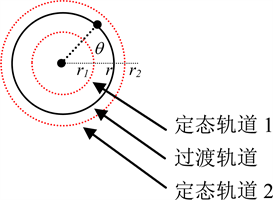Figure 2. The two matter waves of stationary state 1 and 2 coexist in one interim state

$\psi {\psi }^{*}={a}_{1}^{2}+{a}_{2}^{2}+2{a}_{1}{a}_{2}\mathrm{cos}\left(\frac{\left({J}_{2}-{J}_{1}\right)\theta }{\hslash }-\frac{\left({E}_{2}-{E}_{1}\right)t}{\hslash }\right)$ (14)

$\frac{{J}_{2}-{J}_{1}}{\hslash }=k;\text{ }k=1,2,\cdots$ (15)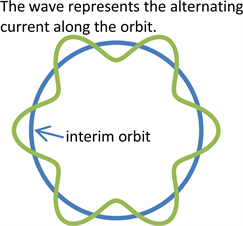Figure 3. The wave on the orbit represents the electron probability in the interim orbit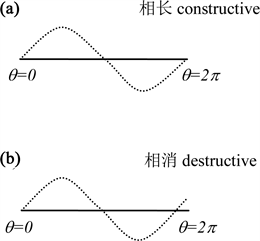Figure 4. The construction of the alternating current on the orbit allows the current to survive in the interim orbit for k being an integer

$\begin{array}{c}j\left(\theta ,t\right)=\frac{\hslash }{2i{m}_{e}}\left({\psi }^{*}\frac{\partial \psi }{r\partial \theta }-\psi \frac{\partial {\psi }^{*}}{r\partial \theta }\right)\\ =\frac{1}{{m}_{e}r}\left[{a}_{1}^{2}{J}_{1}+{a}_{2}^{2}{J}_{2}+{a}_{1}{a}_{2}\left({J}_{2}+{J}_{1}\right)\mathrm{cos}\left(\frac{\left({J}_{2}-{J}_{1}\right)\theta }{\hslash }-\frac{\left({E}_{2}-{E}_{1}\right)t}{\hslash }\right)\right]\end{array}$ (16)

4. 量子跃迁过程中的Rabi振荡：功能转换过程

$E={a}_{1}^{2}{E}_{1}+{a}_{2}^{2}{E}_{2}={E}_{1}+{a}_{2}^{2}\left({E}_{2}-{E}_{1}\right)$ (17)

$\frac{\text{d}E}{\text{d}t}={v}_{\theta }{f}_{\theta }=je{E}_{\theta }=je{E}_{0}\mathrm{cos}\left(\omega t-\theta \right)$ (18)

$\begin{array}{c}\frac{\text{d}E}{\text{d}t}=\frac{e{E}_{0}\left({a}_{1}^{2}{J}_{1}+{a}_{2}^{2}{J}_{2}\right)}{{m}_{e}r}\mathrm{cos}\left(\omega t-\theta \right)\\ \text{\hspace{0.17em}}\text{\hspace{0.17em}}+\frac{e{E}_{0}{a}_{1}{a}_{2}\left({J}_{2}+{J}_{1}\right)}{{m}_{e}r}\mathrm{cos}\left(\frac{\left({J}_{2}-{J}_{1}\right)\theta }{\hslash }-\frac{\left({E}_{2}-{E}_{1}\right)t}{\hslash }+\varphi \right)\mathrm{cos}\left(\omega t-\theta \right)\end{array}$ (19)

$\frac{{J}_{2}-{J}_{1}}{\hslash }=1;\text{ }\frac{{E}_{2}-{E}_{1}}{\hslash }={\omega }_{0}$ (20)

${\frac{\text{d}E}{\text{d}t}|}_{\theta =0}=\frac{e{E}_{0}{a}_{1}{a}_{2}\left({J}_{2}+{J}_{1}\right)}{{m}_{e}r}\mathrm{cos}\left({\omega }_{0}t\right)\mathrm{cos}\left(\omega t\right)$ (21)

${\int }_{0}^{{a}_{2}}\frac{2\left({E}_{2}-{E}_{1}\right)}{\sqrt{1-{a}_{2}^{2}}}\text{d}\left({a}_{2}\right)=\frac{e{E}_{0}\left({J}_{2}+{J}_{1}\right)}{{m}_{e}r}{\int }_{0}^{t}\mathrm{cos}\left({\omega }_{0}t\right)\mathrm{cos}\left(\omega t\right)\text{d}t$ (22)

$\mathrm{arcsin}{a}_{2}=\frac{e{E}_{0}\left({J}_{2}+{J}_{1}\right)}{2\left({E}_{2}-{E}_{1}\right){m}_{e}r}\left[\frac{\mathrm{sin}\left(\left({\omega }_{0}-\omega \right)t\right)}{2\left({\omega }_{0}-\omega \right)}+\frac{\mathrm{sin}\left(\left({\omega }_{0}+\omega \right)t\right)}{2\left({\omega }_{0}+\omega \right)}\right]$ (23)

$\mathrm{arcsin}{a}_{2}=\frac{e{E}_{0}\left({J}_{2}+{J}_{1}\right)}{\text{4}{\omega }_{0}\hslash {m}_{e}r}\frac{\mathrm{sin}\left[\left({\omega }_{0}-\omega \right)t\right]}{{\omega }_{0}-\omega }=\frac{{V}^{\prime }}{2\hslash }\frac{\mathrm{sin}\left[\left({\omega }_{0}-\omega \right)t\right]}{{\omega }_{0}-\omega }$ (24)

$p\left(t\right)={a}_{2}^{2}={\mathrm{sin}}^{2}\left(\frac{{V}^{\prime }}{2\hslash }\frac{\mathrm{sin}\left[\left({\omega }_{0}-\omega \right)t\right]}{{\omega }_{0}-\omega }\right)$ (25)

${a}_{2}=1$ 的时候，量子跃迁结束。如果继续辐照激光，跃迁概率会从1下降，直到零，让后又会增加，如此反复，形成Rabi振荡。

$\frac{e{E}_{0}\left({J}_{2}+{J}_{1}\right)}{\text{2}{\omega }_{0}\hslash {m}_{e}\left({r}_{2}+{r}_{1}\right)}\frac{\mathrm{sin}\left[\left({\omega }_{0}-\omega \right)\tau \right]}{{\omega }_{0}-\omega }=\frac{\text{π}}{\text{2}}$ (26)

$\tau =\frac{1}{{\omega }_{0}-\omega }\mathrm{arcsin}\left(\frac{{\omega }_{0}\hslash {m}_{e}\left({r}_{2}+{r}_{1}\right)\left({\omega }_{0}-\omega \right)}{\text{π}e{E}_{0}\left({J}_{2}+{J}_{1}\right)}\right)$ (27)

$\begin{array}{c}\mathrm{arcsin}{a}_{2}=\frac{{V}^{\prime }}{2\hslash }\frac{\mathrm{sin}\left[\left({\omega }_{0}-\omega \right)t\right]}{{\omega }_{0}-\omega }\\ =\frac{{V}^{\prime }}{\hslash }\frac{\mathrm{sin}\left[\left({\omega }_{0}-\omega \right)t/2\right]}{{\omega }_{0}-\omega }\mathrm{cos}\left[\left({\omega }_{0}-\omega \right)t/2\right]\end{array}$ (28)

$\mathrm{sin}\alpha =2\mathrm{sin}\frac{\alpha }{2}\mathrm{cos}\frac{\alpha }{2}\simeq 2\mathrm{sin}\frac{\alpha }{2}$ (29)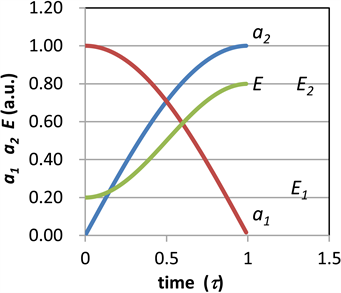Figure 5. The dynamic evolution curve was calculated by computer simulation, when a2 = 1, the quantum transition finished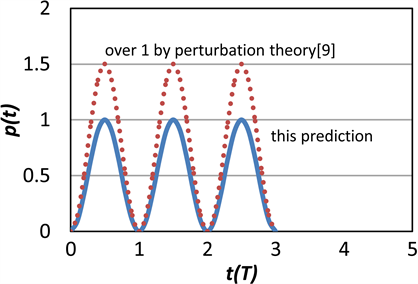Figure 6. Transition probabilities as time function, probability over 1 predicted by perturbation theory (dash line), the real line represents our prediction

${a}_{2}=\frac{{V}^{\prime }}{\hslash }\frac{\mathrm{sin}\left[\left({\omega }_{0}-\omega \right)t/2\right]}{{\omega }_{0}-\omega }$ (30)

$p\left(t\right)={a}_{2}^{2}=\frac{{{V}^{\prime }}^{2}}{{\hslash }^{2}}\frac{{\mathrm{sin}}^{2}\left[\left({\omega }_{0}-\omega \right)t/2\right]}{{\left({\omega }_{0}-\omega \right)}^{2}}$ (31)

${\omega }_{r}=\frac{1}{2}\sqrt{{\left({\omega }_{0}-\omega \right)}^{2}+\frac{{{V}^{\prime }}^{2}}{{\hslash }^{2}}}$ (32)

5. 结论

Relativistic De Broglie Matter Wave and Its Application to Dynamic Rabi Flopping in Quantum Transition[J]. 现代物理, 2018, 08(03): 139-147. https://doi.org/10.12677/MP.2018.83017

1. 1. Gasiorowicz, S. (2003) Quantum Physics. John Wiley & Sons, 5.

2. 2. 曾谨言. 量子力学, 上册[M]. 科学出版社, 1982: 18.

3. 3. 崔怀洋. 相对论的德布罗意物质波及其应用于Aharonov-Bohm效应[J]. 大学物理, 2017, 36(8): 1-4+35.

4. 4. Bohm, D. (1952) A Suggested Interpretation of the Quantum Theory in Terms of Hidden Variables. I. Physical Review, 85, 166-179. https://doi.org/10.1103/PhysRev.85.166

5. 5. Bohm, D. (1952) A Suggested Interpretation of the Quantum Theory in Terms of Hidden Variables. II. Physical Review, 85, 180-193. https://doi.org/10.1103/PhysRev.85.180

6. 6. Einstein, A., Podolsky, B. and Rosen, N. (1935) Can Quantum-Mechanical Description of Physical Reality Be Considered Complete. Physical Review, 47, 777-780. https://doi.org/10.1103/PhysRev.47.777

7. 7. Sanz, A.S. and Borondo, F. (2007) A Quantum Trajectory Description of Decoherence. The European Physical Journal D, 44, 319-326.

8. 8. 沈慧川, 译. 德布罗意文选[M]. 北京大学出版社, 2012.

9. 9. Griffith, D. (2006) Introduction to Quantum Mechanics. 机械工业出版社, 346. (英文影印).

10. 10. Griffith, D. (2006) Introduction to Quantum Mechanics. 机械工业出版社, 348. (英文影印).